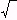edHelper subscribers - Create a new printable

Sample edHelper.com - Conics Worksheet

 Name _____________________________Date ___________________
Conics
Find the distance between P and Q and the coordinates of the midpoint between the two points.
1.
P
 ( -5 , -6 )
and
Q
(
-1
 56
,  -24)

2.
P
 ( 6 , -12 )
and
Q
 ( -12 , -9 )

Find the distance between P and Q and the coordinates of the midpoint between the two points.
3.
P
(
105
,
65
)
and
Q
(
145
,
95
)

4.
P
(
1010
,
82
)
and
Q
(
1810
,
142
)

Point M is the midpoint between the points P and Q. Find the coordinates of the missing point.
5.
Q
(5  ,
-5
 35
)

M
(
8
 711
,
-3
 6790
)

6.
P
 ( -7 , -2 )

M
(-1  ,
 12
)

Point M is the midpoint between the points P and Q. Find the coordinates of the missing point.
7.
P
(
22
,
1010
)

M
(
132

2
,
125
)

8.
P
(
96
,
117
)

M
(
236

2
,
257

2
)

The points A and C are the endpoints of a diameter in a circle. Find the point B which is the center of the circle. Also find the circumference and area of the circle.
9.
A
 ( -9 , 1 )

C
 ( -13 , -7 )

10.
A
 ( -5 , 9 )

C
 ( -18 , -3 )

Write the equation of the parabola in standard form.
11.
y  =
 124
x2

12.
-36x  =
 34
y2

13.
 40y = x2

Find the focus and the directrix of the parabola. The vertex of the parabola is (0,0).
14.
x + 63  =
 144
y2 + 63

15.
 -136
x2  =  y

16.
x  =
 -140
y2

Find the focus, vertex, and the directrix of the parabola.
17.
 -4y2 +  8y +  96x -  868 = 0

18.
y  -  2  =  -
 144
(x  -  8)2

19.
 x2 -  12x -  48y +  276 = 0

Write the equation for the parabola that has the given characteristics.
 20 focus: (1,0)directrix: x=-1
 21 vertex: (-9, 4)focus: (-9, 16)
 22 vertex: (0, 0)directrix: x=4

Write the standard form of the equation of the circle with the given radius and center.
23.
C (0, 0); r =
 13

24.
C (6, 2); r =
12  +7

25.
C (0, 0); r =13

Write the standard equation, the center, and the radius for each circle.
26.
x2 + y2 - 6x + 9  =
 136

27.
x2 + y2 + 6y + 9  =
 116

Write the standard form of the equation of the circle with the given center C that passes through the given point Z.
28.
 C (-4, -9); Z (-4, -4)

29.
 C (0, -7); Z (-2, -7)

30.
 C (3, 8); Z (-7, 9)

Find the foci, vertices, and co-vertices of each ellipse.
31.
 x281
+
 y29
=    1

32.
 x236
+
 y2256
=    1

33.
 x225
+
 y2121
=    1

Find the center, foci, vertices, and co-vertices of each ellipse.
34.
 (x   -  5)216
+
 (y   +  2)236
=    1

35.
 (x   -  1)281
+
 (y   -  7)24
=    1

36.
 (x   +  7)216
+
 (y   +  6)21
=    1

Write the standard equation of each ellipse. Find the center, foci, vertices, and co-vertices of each ellipse.
37.
 36x2 + 25y2 - 216x - 100y - 476 = 0

38.
3x2  +
 3y24
-    3    =    0

Write the standard equation for the ellipse.
 39 Center (0,0)Vertex: (0,-6)Co-Vertex: (3,0)
 40 Center (0,0)Vertex: (0,9)Co-Vertex: (-4,0)
41.   Center (0,0)
Vertex: (2,0)
Focus:
(3
,  0)

Write the standard equation for the ellipse.
42.
Foci:
(3  ,
4 -
42
)
and
(3  ,
4 +
42
)
co-vertices: (1,4) and (5,4)

43.
Foci:
 (0 , -4 )
and
 (0 , 4 )
co-vertices: (-3,0) and (3,0)

Find the vertices, co-vertices, foci, and asymptotes of the hyperbola.
44.
 x2169
-
 y2121
=    1

45.
 y236
-
 x29
=    1

Find the vertices, co-vertices, and foci of the hyperbola.
46.
 (x   +  5)29
-
 (y   +  1)29
=    1

47.
 (y   +  7)216
-
 (x   -  6)281
=    1

Write the equation in standard form.  Find the vertices, co-vertices, and foci of the hyperbola.
48.
 y2 - x2 - 4y - 2x - 78 = 0

49.
 y24
-
 9x225
=    9

Write the standard equation for the hyperbola with the given characteristics.
50.
Foci:
 ( -10 , 0)
and
 ( 10 , 0)
co-vertices: (0,-8) and (0,8)

Write the standard equation for the hyperbola with the given characteristics.
51.   center: (-3,-3)
Foci:
(
-3 -
35
,  -3)
and
(
-3 +
35
,  -3)
co-vertices: (-3,-6) and (-3,0)

Classify the conic section.
52.
 (x   +  2)249
-
 (y   +  3)264
=    1

53.
x  =
 -116
y2

Classify the conic section.  Write the standard equation of the conic section.
54.
 9x2 - 4y2 - 54x - 8y - 247 = 0

55.
x + 44  =
 -148
y2 + 44

Sample
This is only a sample worksheet.

edHelper subscribers - Create a new printable